# Numb3rs 217: Mind Games

The two mathematically significant moments in this episode are the card-color guessing game to which Agent Reeves challenges the psychic and Charlie's use of the Fokker-Planck equation in an attempt to determine where migrants are likely to be found. Both are based on the science of probability.

## Psychic Testing 101

Suppose one day, out of the blue, a friend calls you up and claims to have acquired psychic abilities, like the ability to see things telepathically, without physically observing them. You are naturally curious, and so you decide to test your friend by making him guess the color of cards he can't see, much like Agent Reeves tested the alleged remote-seer in the episode.

To avoid bias and reduce the possibility of cheating, scientists usually conduct double blind trials, where neither the subject nor the experimenter know at the time of experiment which answers are correct and which are not. In your case, you might put the cards in identical envelopes, shuffle them, and only open each envelope following your friend's guess.

The idea of this experiment is that while a good guesser might pick the color correctly once, twice, or even three or four times in a row, after enough correct answers you'll become convinced that something other than guessing is going on. How convinced and at what point? Let's find out.

Activity 1: First we should agree on notation. I'll denote by p(n;m) the probability of a person guessing at random the color of m cards correctly out of a total of n cards. What are the values of the following?
1. p(1;1)
2. p(1;0)
3. p(2;2) (Hint: recall that the probability of two independent events occurring is the product of their probabilities)
4. p(2;1)
5. p(n;n), where n is any positive integer.
6. Is p(n;n)=p(n;0) for every positive integer n?

One way to answer #4 above is to look at the order of choices. Guessing one color correctly and another wrongly can be done by guessing the color of the first card and not of the second (0.5*0.5=0.25) or by missing the color of the first card and correctly identifying the color of the second card (another 0.5*0.5=0.25) for a total probability of 0.5. This case analysis can be generalized if we make one crucial observation. Suppose the cards are put into envelopes which are numbered 1 through n, and suppose further that someone randomly guesses the color of each card. You then open the envelopes and record a sequence of n results, ordered by the numbers on the envelopes. For n=4 for instance, you might get the sequence

` (RIGHT WRONG WRONG RIGHT) `
corresponding to correct guesses for the cards in envelopes 1 and 4, and incorrect guesses for 2 and 3. Since the guesses are independent events, and each has a probability of 0.5 of being correct, each particular ordered sequence of rights and wrongs has an equal probability of occurring as any other, that is 0.5n, for n guesses made. Thus to find p(n;m) all we must do is calculate the number of different ordered sequences of length n with m right guesses and n-m wrong, from now on denoted C(n,m), and multiply it by the probability of each occurring, i.e. 0.5n. Putting all this into one equation,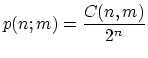Activity 2: What is C(n,m)?
1. Suppose you have before you n white marbles, numbered consecutively from 1 to n. How many different ordered sequences of numbers can you produce by arranging the marbles side by side into an ordered sequence? (Hint: imagine that you start out with n marked spots on which you place the marbles to make your sequence. Then you have n different spots to place the first marble on, n-1 spots to place the second marble, n-2 for the third, and so on.)
2. Now we change the procedure a bit: we color the first m numbered marbles red, and the remaining n-m blue. We also separate the red and blue numbered marbles. How many different pairs of ordered sequences are there corresponding to separate arrangements of red and blue marbles? (Hint: for every ordered arrangement of red marbles, how many different ordered arrangements of blue marbles are there?)

The connection between the above two questions and the value of C(n,m) is the following: to make an ordered sequence of numbered marbled as in #1 above we can first color the marbles one of two colors, as in #2, then arrange the marbles of each colors into two separate colored sequences, and finally put those two sequences together according to a length n sequence of R's and B's corresponding to the number of red and blue marbles (see illustration below).Thus we get that the number of arrangements in #1, n!, is equal to the number of arrangements in #2 times the number of different sequences of length n of m R's and n-m B's, that is C(n,m). And so we arrive at the sought out answer, n!=m!(n-m)!C(n,m), implying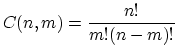Activity 3:
1. What are the values of p(20;8) and p(20;12)? How about p(13;6) and p(13;7)?
2. Can you see a pattern? Why does it occur?
Activity 4:
1. What's the probability of guessing at random the color of 15 or more out of 20 cards?
2. What about 10 or more, out of 20?
3. How about 9 or less?
4. What's the connection between the last two answers?

Now you're ready to test your friend's claims, having at your disposal the ability to tell how likely a particular result is if the guesses are random. How many colors of cards would your friend need to correctly identify, say out of a total of 20, in order to convince you that he's not just randomly guessing?

Despite wide public fascination with psychic phenomena, no one has yet been able to achieve a statistically significant result in tests like the one above (i.e. consistently doing better than random guessing). For the last few decades the magician James Randi has been offering a million dollars for any demonstration of paranormal powers. Nobody has won this prize so far.

## Meet the Fokker-Planck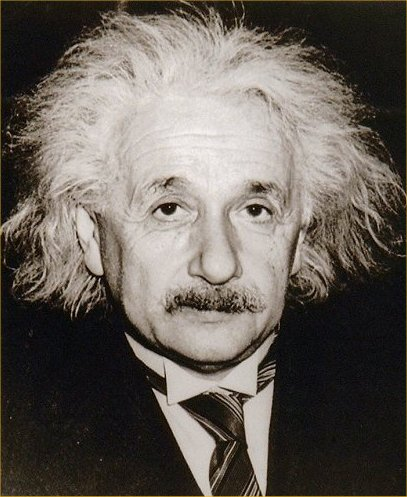Actually, the story begins with neither Fokker nor Planck, but Einstein, who in 1905 published a paper about a very curious phenomenon observed by botanist Robert Brown almost a century earlier. As he was looking at pollen submerged in water under a microscope, Brown noticed tiny particles--much smaller than the pollen grains themselves--moving in seemingly random manner in the water. He confirmed that this motion did not depend on the type of particles submerged, just on their minuscule size. The science of the time could not explain this; the missing ingredient was the atomic and molecular model of matter, developed only toward the end of the 19th century

Armed with the newly developed molecular theory of matter, Einstein proposed the following simple solution: the fact that the water has positive temperature just means that water molecules are moving around, and if the submerged particle is small enough, it gets pushed around by the molecules.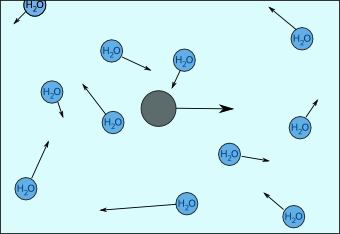Consider a top-down view of a small particle of mass m immersed in water, moving with some velocity v(t) at time t, as in the picture below. We ignore the force of gravity pulling it down and buoyancy pushing it up; we're only interested in motion in the horizontal plane. In this case, the only force acting on the particle is drag, the friction force of water. This force is proportional to the velocity v, that is,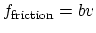for some positive constant b depending on the shape of the particle. Thus, by Newton's second law of motion, we have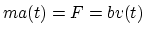where a(t) is the acceleration at time t. Since acceleration is just the change in velocity, we get the equation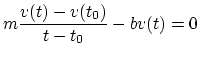Activity 1: Suppose our particle starts out at time t=0 moving North with velocity v(0), with no external force acting on it. Why do the following velocity equations v(t) fail to fit our model?
1. v(t)=cos(t)/t
2. v(t)=3t2+t+1
3. v(t)=5-t for 0≤t≤5, and v(t)=0 for t≥5.
Solving the equationfor v(t) as t-t0 becomes small, we get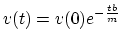Activity 2*: If you know calculus, first show that this solution fits the equation, then derive it yourself.
Activity 3: Suppose m=8, b=3 and the velocity at time zero is v(0)=5m/s. When will the velocity decrease to less than 1m/s? Less than 0.1m/s?
Thus as we expect, the speed of the particle decreases to zero. If the particle is relatively massive then this is the end of the story: given the initial velocity, you can calculate where the particle will be at time t and its velocity at that time. However, if the particle is small enough, it will get pushed around by water molecules bumping into it, and the above description is no longer accurate.

Given a small enough particle, each collision with a water molecule will result in a transfer of momentum. That is, the colliding molecule will exert a force on our particle for the very short duration of collision, leading to a change in the particle's velocity. Since thousands such collisions occur each second, it's practically impossible to account for the movement of every molecule and solve the resulting system of equations to find out what exact effect the collisions will have on our particle. Thus we do the next best thing: approximate this effect by a "random" fluctuating force F(t), so our equation becomes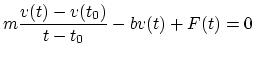The Fokker-Plank equation is based on a generalization of this assumption, where given data about what the random component of the motion is like (called diffusion term), and knowing the few non-random forces acting on the particle (the drift terms), the equation allows you to figure out what is the probability of a particle being in a certain area at a future time. This idea can be applied to any situation in which a quantity, for instance the population of parrots on an island, is subject to known deterministic forces--availability of food, presence of predators--and smaller, but significant "randomly fluctuating" forces--like weather pattern changes for instance.